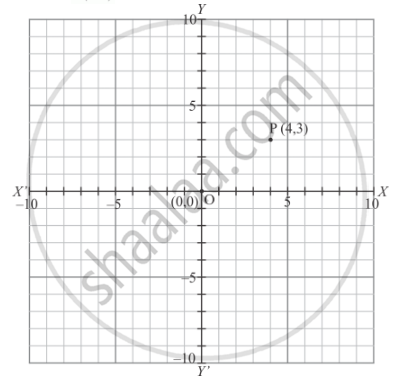# The perpendicular distance of the P (4,3) from y-axis is - Mathematics

MCQ

The perpendicular distance of the P (4,3)  from y-axis is

#### Options

• 4

• 3

• 5

• none of these

#### Solution

The point  P(4,3) is shown in the graph given below:Thus the perpendicular distance of the point  P(4,3) from y−axis is 4.

Concept: Coordinate Geometry
Is there an error in this question or solution?

#### APPEARS IN

RD Sharma Mathematics for Class 9
Chapter 8 Co-ordinate Geometry
Exercise 8.2 | Q 12 | Page 7

Share